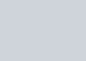A line is perpendicular to two lines having direction ratios
Question:

A line is perpendicular to two lines having direction ratios $1,-2,-2$ and $0,2,1$. The direction cosines of the line are

A. $\frac{-2}{3}, \frac{1}{3}, \frac{2}{3}$

B. $\frac{2}{3}, \frac{1}{3}, \frac{-1}{3}$

C. $\frac{2}{3}, \frac{-1}{3}, \frac{2}{3}$

D. none of these

Solution: